# SB Audience

With well over 35 years of experience in development and manufacturing of solutions for professional OEM drivers, the time is ripe to bring the own brand to the world market.

SB Audience is spawned from the heavyweight manufacturing of Sinar Baja Electric Indonesia and has serviced well known brands in the PRO audio industry for decades with in house engineering and manufacturing. There are three categories within the standard product range: Bianco, achieves good performance at the most reasonable cost. Rosso, aims at high performance at an attractive price, and Nero is the higher performance line still achieving exceptional price performance ratio.

If you don't find what you are looking for, SB Audience has the engineering capabilities and the manufacturing capacity to meet a wide variety of needs.

## Compression driver for Horns## SB Audience BIANCO-25CD-P

order no. sba-b-25CD-P

EUR 24,80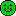excl. VAT: € 20.84 / \$ 22.30

1 inch high frequency compression driver with polyetherimide cone.

• power handling (continuous/programme) = 20/40 W
• frequency range = 1000-20000 Hz
• impedance R = 8 Ohm
• sound pressure level SPL = 109 dB (1W; 1m)
• DC resistance Re = 5,5 Ohm
• force factor BL = 1,7 N/A
• total Q factor Qts = - (Qms=, Qes=)
• voice coil diameter = 25 mm
• maximum peak linear excursion vibration xlin = +/- 0,2 mm
• mounting diameter d = 25,4 mm
• overall diameter d = 90 mm
• mounting depth (not countersunk) t = 56,3 mm
• mass m = 0,84 kg
PDF data sheet

## SB Audience BIANCO-34CD-T

order no. sba-b-34CD-T

EUR 55,30excl. VAT: € 46.47 / \$ 49.72

1 inch high frequency compression driver with titanium cone.

• power handling (continuous/programme) = 30/60 W
• frequency range = 700-20000 Hz
• impedance R = 8 Ohm
• sound pressure level SPL = 108 dB (1W; 1m)
• DC resistance Re = 6 Ohm
• force factor BL = 1,5 N/A
• total Q factor Qts = - (Qms=, Qes=)
• voice coil diameter = 34 mm
• maximum peak linear excursion vibration xlin = +/- 0,4 mm
• mounting diameter d = 25,4 mm
• overall diameter d = 90 mm
• mounting depth (not countersunk) t = 43,9 mm
• mass m = 0,92 kg
PDF data sheet

## SB Audience BIANCO-34CD-PK

order no. sba-b-34CD-PK

EUR 58,10excl. VAT: € 48.82 / \$ 52.24

1 inch high frequency compression driver with high quality cone.

• power handling (continuous/programme) = 30/60 W
• frequency range = 1000-20000 Hz
• impedance R = 8 Ohm
• sound pressure level SPL = 107 dB (1W; 1m)
• DC resistance Re = 6 Ohm
• force factor BL = 1,2 N/A
• total Q factor Qts = - (Qms=, Qes=)
• voice coil diameter = 34 mm
• maximum peak linear excursion vibration xlin = +/- 0,4 mm
• mounting diameter d = 25,4 mm
• overall diameter d = 90 mm
• mounting depth (not countersunk) t = 44,85 mm
• mass m = 0,92 kg
PDF data sheet

## SB Audience BIANCO-44CD-T

order no. sba-b-44CD-T

EUR 61,40excl. VAT: € 51.60 / \$ 55.21

1 inch high frequency compression driver with titanium cone.

• power handling (continuous/programme) = 50/100 W
• frequency range = 700-20000 Hz
• impedance R = 8 Ohm
• sound pressure level SPL = 107 dB (1W; 1m)
• DC resistance Re = 6,2 Ohm
• force factor BL = 1,6 N/A
• total Q factor Qts = - (Qms=, Qes=)
• voice coil diameter = 44 mm
• maximum peak linear excursion vibration xlin = +/- 0,5 mm
• mounting diameter d = 25,4 mm
• overall diameter d = 115 mm
• mounting depth (not countersunk) t = 53,8 mm
• mass m = 1,69 kg
PDF data sheet

## SB Audience BIANCO-44CD-K

order no. sba-b-44CD-K

EUR 72,40excl. VAT: € 60.84 / \$ 65.10

1 inch high frequency compression driver with high quality cone.

• power handling (continuous/programme) = 50/100 W
• frequency range = 700-20000 Hz
• impedance R = 8 Ohm
• sound pressure level SPL = 110 dB (1W; 1m)
• DC resistance Re = 6,2 Ohm
• force factor BL = 1,6 N/A
• total Q factor Qts = - (Qms=, Qes=)
• voice coil diameter = 44 mm
• maximum peak linear excursion vibration xlin = +/- 0,5 mm
• mounting diameter d = 25,4 mm
• overall diameter d = 120 mm
• mounting depth (not countersunk) t = 61,46 mm
• mass m = 2,22 kg
PDF data sheet

## SB Audience BIANCO-44CD-PK

order no. sba-b-44CD-PK

EUR 73,30excl. VAT: € 61.60 / \$ 65.91

1 inch high frequency compression driver with high quality cone.

• power handling (continuous/programme) = 50/100 W
• frequency range = 700-20000 Hz
• impedance R = 8 Ohm
• sound pressure level SPL = 107 dB (1W; 1m)
• DC resistance Re = 6,2 Ohm
• force factor BL = 1,6 N/A
• total Q factor Qts = - (Qms=, Qes=)
• voice coil diameter = 44 mm
• maximum peak linear excursion vibration xlin = +/- 0,5 mm
• mounting diameter d = 25,4 mm
• overall diameter d = 120 mm
• mounting depth (not countersunk) t = 61,46 mm
• mass m = 2,22 kg
PDF data sheet

## SB Audience CD ROSSO-34CD-PK

order no. sba-CD-ROSSO-34CD-PK

EUR 61,50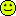excl. VAT: € 51.68 / \$ 55.30

1 inch high frequency compression driver with high quality cone.

• power handling (continuous/programme) = 30/60 W
• frequency range = 1000-20000 Hz
• impedance R = 8 Ohm
• sound pressure level SPL = 105 dB (1W; 1m)
• DC resistance Re = 6 Ohm
• force factor BL = 1,3 N/A
• total Q factor Qts = - (Qms=, Qes=)
• voice coil diameter = 34 mm
• maximum peak linear excursion vibration xlin = +/- 0,5 mm
• mounting diameter d = 25,4 mm
• overall diameter d = 90 mm
• mounting depth (not countersunk) t = 45,86 mm
• mass m = 0,94 kg
PDF data sheet

## SB Audience CD ROSSO-34CDN-PK

order no. sba-CD-ROSSO-34CDN-PK

EUR 88,40excl. VAT: € 74.29 / \$ 79.49

1 inch high frequency compression driver with high quality cone.

• power handling (continuous/programme) = 30/60 W
• frequency range = 1000-20000 Hz
• impedance R = 8 Ohm
• sound pressure level SPL = 106 dB (1W; 1m)
• DC resistance Re = 6 Ohm
• force factor BL = 1,55 N/A
• total Q factor Qts = - (Qms=, Qes=)
• voice coil diameter = 34 mm
• maximum peak linear excursion vibration xlin = +/- 0,5 mm
• mounting diameter d = 25,4 mm
• overall diameter d = 94,5 mm
• mounting depth (not countersunk) t = 53,86 mm
• mass m = 0,55 kg
PDF data sheet

## SB Audience CD ROSSO-44CD-PK

order no. sba-CD-ROSSO-44CD-PK

EUR 111,50excl. VAT: € 93.70 / \$ 100.26

1 inch high frequency compression driver with high quality cone.

• power handling (continuous/programme) = 60/120 W
• frequency range = 700-20000 Hz
• impedance R = 8 Ohm
• sound pressure level SPL = 107 dB (1W; 1m)
• DC resistance Re = 6,2 Ohm
• force factor BL = 1,59 N/A
• total Q factor Qts = - (Qms=, Qes=)
• voice coil diameter = 44 mm
• maximum peak linear excursion vibration xlin = +/- 0,1 mm
• mounting diameter d = 25,4 mm
• overall diameter d = 120 mm
• mounting depth (not countersunk) t = 62,02 mm
• mass m = 2,29 kg
PDF data sheet

## SB Audience CD ROSSO-44CDN-PK

order no. sba-CD-ROSSO-44CDN-PK

EUR 192,50excl. VAT: € 161.76 / \$ 173.09

1 inch high frequency compression driver with high quality cone.

• power handling (continuous/programme) = 60/120 W
• frequency range = 700-20000 Hz
• impedance R = 8 Ohm
• sound pressure level SPL = 109 dB (1W; 1m)
• DC resistance Re = 6,2 Ohm
• force factor BL = 1,85 N/A
• total Q factor Qts = - (Qms=, Qes=)
• voice coil diameter = 44 mm
• maximum peak linear excursion vibration xlin = +/- 0,1 mm
• mounting diameter d = 25,4 mm
• overall diameter d = 100 mm
• mounting depth (not countersunk) t = 49,9 mm
• mass m = 1,34 kg
PDF data sheet

## SB Audience CD ROSSO-65CD-T

order no. sba-CD-ROSSO-65CD-T

EUR 206,00excl. VAT: € 173.11 / \$ 185.23

1.4 inch high frequency compression driver with titanium cone.

• power handling (continuous/programme) = 70/140 W
• frequency range = 500-20000 Hz
• impedance R = 8 Ohm
• sound pressure level SPL = 110 dB (1W; 1m)
• DC resistance Re = 6,2 Ohm
• force factor BL = 1,6 N/A
• total Q factor Qts = - (Qms=, Qes=)
• voice coil diameter = 65 mm
• maximum peak linear excursion vibration xlin = +/- 0,5 mm
• mounting diameter d = 35,6 mm
• overall diameter d = 156 mm
• mounting depth (not countersunk) t = 65,94 mm
• mass m = 3,95 kg
PDF data sheet

## SB Audience CD ROSSO-65CDN-T

order no. sba-CD-ROSSO-65CDN-T

EUR 263,00excl. VAT: € 221.01 / \$ 236.48

1.4 inch high frequency compression driver with titanium cone.

• power handling (continuous/programme) = 70/140 W
• frequency range = 500-20000 Hz
• impedance R = 8 Ohm
• sound pressure level SPL = 112 dB (1W; 1m)
• DC resistance Re = 6,2 Ohm
• force factor BL = 1,9 N/A
• total Q factor Qts = - (Qms=, Qes=)
• voice coil diameter = 65 mm
• maximum peak linear excursion vibration xlin = +/- 0,4 mm
• mounting diameter d = 35,6 mm
• overall diameter d = 120 mm
• mounting depth (not countersunk) t = 53,94 mm
• mass m = 2 kg
PDF data sheet

## SB Audience BIANCO-75CD-T

order no. sba-b-75CD-T

EUR 206,00excl. VAT: € 173.11 / \$ 185.23

1.4 inch high frequency compression driver with titanium cone.

• power handling (continuous/programme) = 100/200 W
• frequency range = 500-22000 Hz
• impedance R = 8 Ohm
• sound pressure level SPL = 111 dB (1W; 1m)
• DC resistance Re = 7 Ohm
• force factor BL = 1,65 N/A
• total Q factor Qts = - (Qms=, Qes=)
• voice coil diameter = 74,5 mm
• maximum peak linear excursion vibration xlin = +/- 0,7 mm
• mounting diameter d = 35,6 mm
• overall diameter d = 170 mm
• mounting depth (not countersunk) t = 65,7 mm
• mass m = 4,42 kg
PDF data sheet

## Horn## SB Audience HORN H225

order no. sba-h-H225

EUR 14,40excl. VAT: € 12.10 / \$ 12.95

Horn for a compression driver with 1 inch throat.

• Throat diameter = 1 inch
• nominal angle (HxV) = 80x50 Deg
• Cut off frequency : 600 Hz
• over all dimensions (HxWxD) = 223,5x223,5x161 mm
• baffle cutout dimensions (HxW) = 181x184,5 mm
• material: synthetics ABS
PDF data sheet

## SB Audience HORN H250

order no. sba-h-H250

EUR 18,00excl. VAT: € 15.13 / \$ 16.18

Horn for a compression driver with 1 inch throat.

• Throat diameter = 1 inch
• nominal angle (HxV) = 90x90 Deg
• Cut off frequency : 600 Hz
• over all dimensions (HxWxD) = 250x250x149 mm
• baffle cutout dimensions (HxW) = 215x215 mm
• material: synthetics ABS
PDF data sheet

## SB Audience HORN H280

order no. sba-h-H280

EUR 24,20excl. VAT: € 20.34 / \$ 21.76

Horn for a compression driver with 1,4 inch throat.

• Throat diameter = 1,4 inch
• nominal angle (HxV) = 60x40 Deg
• Cut off frequency : 500 Hz
• over all dimensions (HxWxD) = 279,3x279,3x230 mm
• baffle cutout dimensions (HxW) = 239,3x242,8 mm
• material: synthetics ABS
PDF data sheet

## Full Range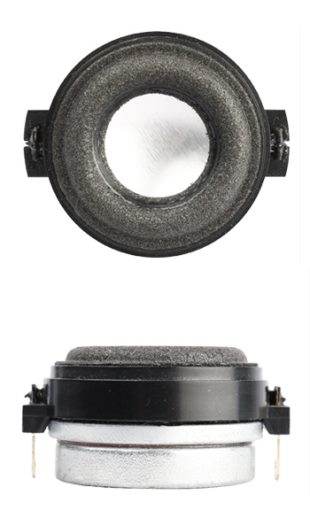open a bigger photo

recommended cabinet 1:
closed cabinet with 0,01 L volume
from 392/248 Hz (-3dB/-8dB)

recommended cabinet 2:
0,02 L volume bass reflex cabinet
with 2cm-Rohr reflex tube, 107 cm long.
from 193/156 Hz (-3dB/-8dB).

## SB Audience SB36WBAC21-4 (1 inch)

order no. sba-SB36WBAC21-4

EUR 20,50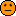excl. VAT: € 17.23 / \$ 18.43

4 cm full range driver with high quality cone.

• power handling (continuous/programme) = 5 W
• frequency range = 250-20000 Hz
• resonance frequency fs = 200 Hz
• impedance R = 4 Ohm
• sound pressure level SPL = 77 dB (2,83V; 1m)
• DC resistance Re = 3,4 Ohm
• force factor BL = 1,76 N/A
• voice coil inductance L = 0,12 mH
• effective piston radiating area Sd = 4,2 cm2
• effective mechanical mass incl. air load mms = 0,76 g
• equivalent volume of compliance Vas = 0,021 l
• total Q factor Qts = 0,41 (Qms=0,68, Qes=1,05)
• voice coil diameter = 20,4 mm
• maximum peak linear excursion vibration xlin = +/- 1,75 mm
• mounting diameter d = 34 mm
• overall diameter d = 43 mm
• mounting depth (not countersunk) t = 20,8 mm
• mass m = 0,055 kg
PDF data sheet

## SB Audience SB36WBAC21-8 (1 inch)

order no. sba-SB36WBAC21-8

EUR 20,50excl. VAT: € 17.23 / \$ 18.43

4 cm full range driver with high quality cone.

• power handling (continuous/programme) = 5 W
• frequency range = 250-20000 Hz
• resonance frequency fs = 160 Hz
• impedance R = 8 Ohm
• sound pressure level SPL = 75 dB (2,83V; 1m)
• DC resistance Re = 5,8 Ohm
• force factor BL = 2,1 N/A
• voice coil inductance L = 0,13 mH
• effective piston radiating area Sd = 4,2 cm2
• effective mechanical mass incl. air load mms = 0,7 g
• equivalent volume of compliance Vas = 0,035 l
• total Q factor Qts = 0,6 (Qms=1,75, Qes=0,92)
• voice coil diameter = 20,4 mm
• maximum peak linear excursion vibration xlin = +/- 1,75 mm
• mounting diameter d = 34 mm
• overall diameter d = 43 mm
• mounting depth (not countersunk) t = 20,8 mm
• mass m = 0,055 kg
PDF data sheet

## SB Audience SB65WBAC25-4 (2,5 inch)

order no. sba-SB65WBAC25-4

EUR 32,00excl. VAT: € 26.89 / \$ 28.77

7 cm full range driver with high quality cone.

• power handling (continuous/programme) = 20 W
• frequency range = 100-20000 Hz
• resonance frequency fs = 115 Hz
• impedance R = 4 Ohm
• sound pressure level SPL = 83,5 dB (2,83V; 1m)
• DC resistance Re = 3,6 Ohm
• force factor BL = 2,9 N/A
• voice coil inductance L = 0,15 mH
• effective piston radiating area Sd = 20 cm2
• effective mechanical mass incl. air load mms = 2,5 g
• equivalent volume of compliance Vas = 0,43 l
• total Q factor Qts = 0,68 (Qms=6, Qes=0,77)
• voice coil diameter = 20,4 mm
• maximum peak linear excursion vibration xlin = +/- 1,75 mm
• mounting diameter d = 64 mm
• overall diameter d = 80 mm
• mounting depth (not countersunk) t = 38 mm
• mass m = 0,055 kg
PDF data sheet

## SB Audience SB10PGC21-4 (3 inch)

order no. sba-SB10PGC21-4

EUR 14,50excl. VAT: € 12.18 / \$ 13.04

9 cm full range driver with high quality cone.

• power handling (continuous/programme) = 20 W
• frequency range = 90-20000 Hz
• resonance frequency fs = 89 Hz
• impedance R = 4 Ohm
• sound pressure level SPL = 84 dB (2,83V; 1m)
• DC resistance Re = 3,4 Ohm
• force factor BL = 2,3 N/A
• voice coil inductance L = 0,15 mH
• effective piston radiating area Sd = 27 cm2
• effective mechanical mass incl. air load mms = 2,8 g
• equivalent volume of compliance Vas = 1,2 l
• total Q factor Qts = 0,93 (Qms=11,2, Qes=1,01)
• voice coil diameter = 20,4 mm
• maximum peak linear excursion vibration xlin = +/- 2,25 mm
• mounting diameter d = 76 mm
• overall diameter d = 95,5 mm
• mounting depth (not countersunk) t = 50,8 mm
• mass m = 0,24 kg
PDF data sheet

## Woofer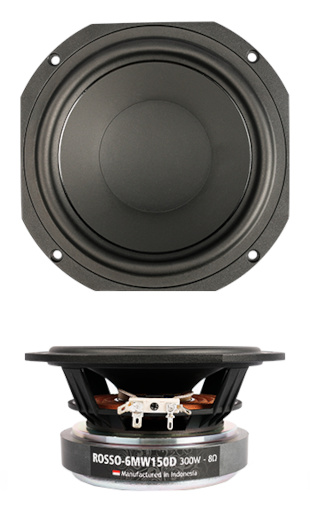open a bigger photo

recommended cabinet 1:
closed cabinet with 1,4 L volume
from 165/104 Hz (-3dB/-8dB)

recommended cabinet 2:
4,4 L volume bass reflex cabinet
with HP50 reflex tube, 14 cm long.
from 89/69 Hz (-3dB/-8dB).

## SB Audience ROSSO-6MW150D

order no. sba-r-6MW150D

EUR 108,00excl. VAT: € 90.76 / \$ 97.11

17 cm bass driver with specially treated paper cone. The basket is made of die-cast.

• power handling (continuous/programme) = 150/300 W
• frequency range = 66-5000 Hz
• resonance frequency fs = 66 Hz
• impedance R = 8 Ohm
• sound pressure level SPL = 94 dB (1W; 1m)
• DC resistance Re = 5,4 Ohm
• force factor BL = 11,6 N/A
• voice coil inductance L = 0,39 mH
• effective piston radiating area Sd = 145,3 cm2
• effective mechanical mass incl. air load mms = 19,4 g
• equivalent volume of compliance Vas = 8,8 l
• total Q factor Qts = 0,32 (Qms=9,24, Qes=0,33)
• voice coil diameter = 49,5 mm
• maximum peak linear excursion vibration xlin = +/- 3,6 mm
• mounting diameter d = 147,5 mm
• overall diameter d = 178 mm
• mounting depth (not countersunk) t = 84 mm
• mass m = 2,62 kg
PDF data sheet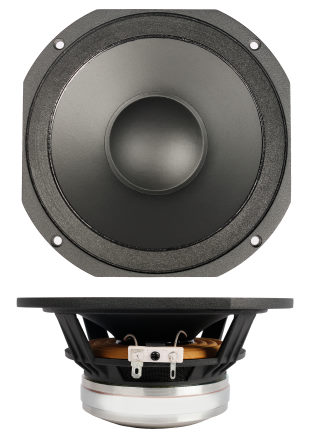open a bigger photo

recommended cabinet 1:
closed cabinet with 0,54 L volume
from 330/208 Hz (-3dB/-8dB)

recommended cabinet 2:
2,2 L volume bass reflex cabinet
with HP70 reflex tube, 12 cm long.
from 154/129 Hz (-3dB/-8dB).

## SB Audience NERO-6MRN150D

order no. sba-n-6MRN150D

EUR 217,00excl. VAT: € 182.35 / \$ 195.12

17 cm midrange driver with specially treated paper cone. The basket is made of die-cast.

• power handling (continuous/programme) = 150/300 W
• frequency range = 200-10600 Hz
• resonance frequency fs = 200 Hz
• impedance R = 8 Ohm
• sound pressure level SPL = 98 dB (1W; 1m)
• DC resistance Re = 5,6 Ohm
• force factor BL = 13,1 N/A
• voice coil inductance L = 0,28 mH
• effective piston radiating area Sd = 136,8 cm2
• effective mechanical mass incl. air load mms = 13,8 g
• equivalent volume of compliance Vas = 1,1 l
• total Q factor Qts = 0,48 (Qms=2,96, Qes=0,58)
• voice coil diameter = 49,5 mm
• maximum peak linear excursion vibration xlin = +/- 2,5 mm
• mounting diameter d = 147,5 mm
• overall diameter d = 178 mm
• mounting depth (not countersunk) t = 73 mm
• mass m = 1,6 kg
PDF data sheet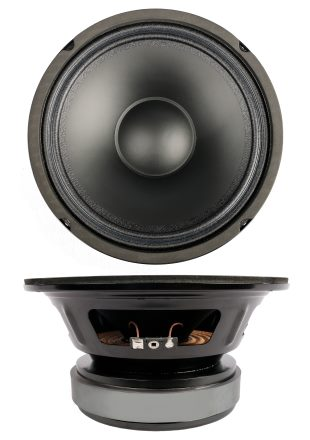open a bigger photo

recommended cabinet 1:
closed cabinet with 7,7 L volume
from 129/81 Hz (-3dB/-8dB)

recommended cabinet 2:
31 L volume bass reflex cabinet
with HP70 reflex tube, 3 cm long.
from 58/50 Hz (-3dB/-8dB).

## SB Audience BIANCO-8MW125

order no. sba-b-8MW125

EUR 60,50excl. VAT: € 50.84 / \$ 54.40

20 cm bass driver with specially treated paper cone.

• power handling (continuous/programme) = 125/250 W
• frequency range = 89-7400 Hz
• resonance frequency fs = 89 Hz
• impedance R = 8 Ohm
• sound pressure level SPL = 97 dB (1W; 1m)
• DC resistance Re = 5,8 Ohm
• force factor BL = 11,3 N/A
• voice coil inductance L = 0,5 mH
• effective piston radiating area Sd = 224,3 cm2
• effective mechanical mass incl. air load mms = 22,8 g
• equivalent volume of compliance Vas = 10,1 l
• total Q factor Qts = 0,55 (Qms=14,63, Qes=0,57)
• voice coil diameter = 49,5 mm
• maximum peak linear excursion vibration xlin = +/- 5 mm
• mounting diameter d = 179,6 mm
• overall diameter d = 205,6 mm
• mounting depth (not countersunk) t = 99 mm
• mass m = 3,47 kg
PDF data sheet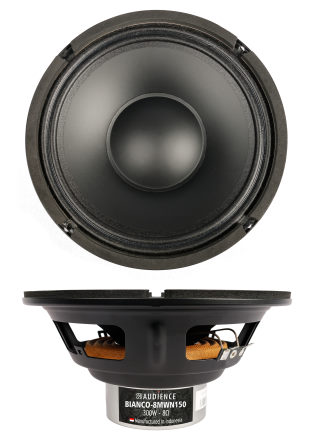open a bigger photo

recommended cabinet 1:
closed cabinet with 2,5 L volume
from 152/96 Hz (-3dB/-8dB)

recommended cabinet 2:
9,4 L volume bass reflex cabinet
with HP70 reflex tube, 14 cm long.
from 76/61 Hz (-3dB/-8dB).

## SB Audience BIANCO-8MWN150

order no. sba-b-8MWN150

EUR 132,00excl. VAT: € 110.92 / \$ 118.69

20 cm bass driver with specially treated paper cone.

• power handling (continuous/programme) = 150/300 W
• frequency range = 74-6000 Hz
• resonance frequency fs = 74 Hz
• impedance R = 8 Ohm
• sound pressure level SPL = 93 dB (1W; 1m)
• DC resistance Re = 6,8 Ohm
• force factor BL = 16,5 N/A
• voice coil inductance L = 1,07 mH
• effective piston radiating area Sd = 227 cm2
• effective mechanical mass incl. air load mms = 34 g
• equivalent volume of compliance Vas = 9,9 l
• total Q factor Qts = 0,38 (Qms=8,97, Qes=0,4)
• voice coil diameter = 60,5 mm
• maximum peak linear excursion vibration xlin = +/- 4,5 mm
• mounting diameter d = 179,6 mm
• overall diameter d = 205,6 mm
• mounting depth (not countersunk) t = 91 mm
• mass m = 1,43 kg
PDF data sheet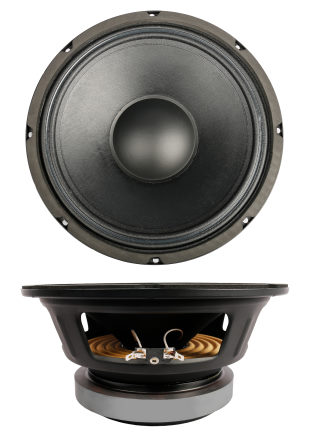open a bigger photo

recommended cabinet 1:
closed cabinet with 6,6 L volume
from 134/85 Hz (-3dB/-8dB)

recommended cabinet 2:
24 L volume bass reflex cabinet
with HP100 reflex tube, 13 cm long.
from 67/54 Hz (-3dB/-8dB).

## SB Audience BIANCO-10MW150

order no. sba-b-10MW150

EUR 81,80excl. VAT: € 68.74 / \$ 73.55

25 cm bass driver with specially treated paper cone.

• power handling (continuous/programme) = 150/300 W
• frequency range = 65-5000 Hz
• resonance frequency fs = 65 Hz
• impedance R = 8 Ohm
• sound pressure level SPL = 100 dB (1W; 1m)
• DC resistance Re = 5,1 Ohm
• force factor BL = 15,1 N/A
• voice coil inductance L = 0,64 mH
• effective piston radiating area Sd = 366,4 cm2
• effective mechanical mass incl. air load mms = 44,4 g
• equivalent volume of compliance Vas = 25,8 l
• total Q factor Qts = 0,39 (Qms=12,08, Qes=0,4)
• voice coil diameter = 60,5 mm
• maximum peak linear excursion vibration xlin = +/- 3 mm
• mounting diameter d = 229 mm
• overall diameter d = 258 mm
• mounting depth (not countersunk) t = 114 mm
• mass m = 4,9 kg
PDF data sheet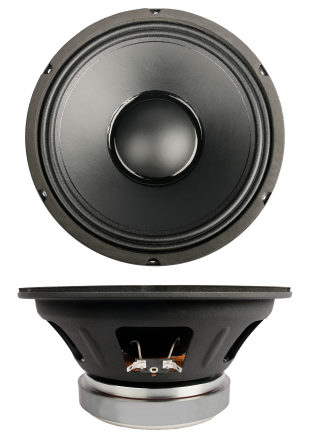open a bigger photo

recommended cabinet 1:
closed cabinet with 8,6 L volume
from 125/79 Hz (-3dB/-8dB)

recommended cabinet 2:
30 L volume bass reflex cabinet
with HP100 reflex tube, 12 cm long.
from 64/51 Hz (-3dB/-8dB).

## SB Audience BIANCO-10MW200

order no. sba-b-10MW200

EUR 81,40excl. VAT: € 68.40 / \$ 73.19

25 cm bass driver with specially treated paper cone.

• power handling (continuous/programme) = 250/500 W
• frequency range = 56-7100 Hz
• resonance frequency fs = 56 Hz
• impedance R = 8 Ohm
• sound pressure level SPL = 98 dB (1W; 1m)
• DC resistance Re = 5,6 Ohm
• force factor BL = 13 N/A
• voice coil inductance L = 0,6 mH
• effective piston radiating area Sd = 366,4 cm2
• effective mechanical mass incl. air load mms = 38 g
• equivalent volume of compliance Vas = 41 l
• total Q factor Qts = 0,36 (Qms=2,28, Qes=0,43)
• voice coil diameter = 60,5 mm
• maximum peak linear excursion vibration xlin = +/- 3,5 mm
• mounting diameter d = 230 mm
• overall diameter d = 257 mm
• mounting depth (not countersunk) t = 110,5 mm
• mass m = 4,4 kg
PDF data sheet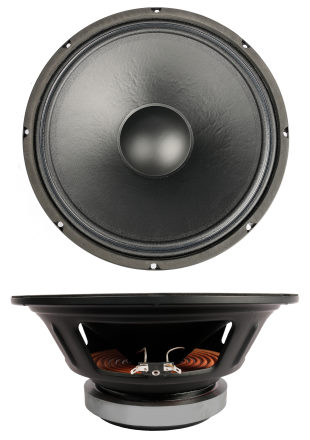open a bigger photo

recommended cabinet 1:
closed cabinet with 33 L volume
from 82/52 Hz (-3dB/-8dB)

recommended cabinet 2:
134 L volume bass reflex cabinet
with 2x HP100 reflex tube, 8 cm long.
from 38/32 Hz (-3dB/-8dB).

## SB Audience BIANCO-12MW200

order no. sba-b-12MW200

EUR 95,00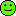excl. VAT: € 79.83 / \$ 85.42

30 cm bass driver with specially treated paper cone.

• power handling (continuous/programme) = 200/400 W
• frequency range = 51-5350 Hz
• resonance frequency fs = 51 Hz
• impedance R = 8 Ohm
• sound pressure level SPL = 99 dB (1W; 1m)
• DC resistance Re = 5,3 Ohm
• force factor BL = 14,7 N/A
• voice coil inductance L = 0,83 mH
• effective piston radiating area Sd = 543,3 cm2
• effective mechanical mass incl. air load mms = 65,2 g
• equivalent volume of compliance Vas = 62,9 l
• total Q factor Qts = 0,49 (Qms=14,33, Qes=0,51)
• voice coil diameter = 60,5 mm
• maximum peak linear excursion vibration xlin = +/- 4,5 mm
• mounting diameter d = 278 mm
• overall diameter d = 306 mm
• mounting depth (not countersunk) t = 126 mm
• mass m = 5,05 kg
PDF data sheet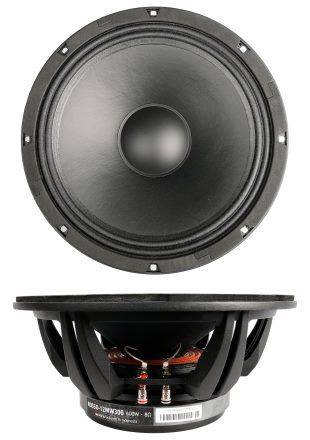open a bigger photo

recommended cabinet 1:
closed cabinet with 9,4 L volume
from 141/89 Hz (-3dB/-8dB)

recommended cabinet 2:
25 L volume bass reflex cabinet
with 2x HP100 reflex tube, 25 cm long.
from 82/62 Hz (-3dB/-8dB).

## SB Audience ROSSO-12MW300

order no. sba-r-12MW300

EUR 172,00excl. VAT: € 144.54 / \$ 154.66

31 cm bass driver with specially treated paper cone. The basket is made of die-cast.

• power handling (continuous/programme) = 300/600 W
• frequency range = 48-5160 Hz
• resonance frequency fs = 48 Hz
• impedance R = 8 Ohm
• sound pressure level SPL = 100 dB (1W; 1m)
• DC resistance Re = 5,2 Ohm
• force factor BL = 17,4 N/A
• voice coil inductance L = 0,73 mH
• effective piston radiating area Sd = 543,3 cm2
• effective mechanical mass incl. air load mms = 54,3 g
• equivalent volume of compliance Vas = 85,9 l
• total Q factor Qts = 0,27 (Qms=12,1, Qes=0,28)
• voice coil diameter = 75,6 mm
• maximum peak linear excursion vibration xlin = +/- 3,5 mm
• mounting diameter d = 289 mm
• overall diameter d = 322,5 mm
• mounting depth (not countersunk) t = 125,3 mm
• mass m = 7,6 kg
PDF data sheet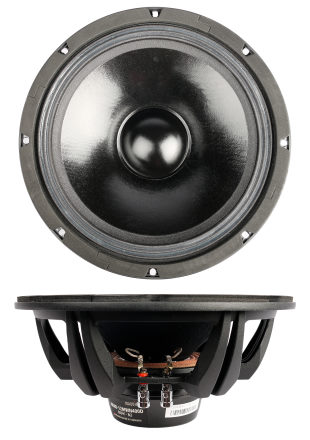open a bigger photo

recommended cabinet 1:
closed cabinet with 20 L volume
from 101/64 Hz (-3dB/-8dB)

recommended cabinet 2:
80 L volume bass reflex cabinet
with 2x HP100 reflex tube, 10 cm long.
from 46/39 Hz (-3dB/-8dB).

## SB Audience NERO-12MWN400D

order no. sba-n-12MWN400D

EUR 249,00excl. VAT: € 209.24 / \$ 223.89

31 cm bass driver with specially treated paper cone. The basket is made of die-cast.

• power handling (continuous/programme) = 400/800 W
• frequency range = 66-6100 Hz
• resonance frequency fs = 66 Hz
• impedance R = 8 Ohm
• sound pressure level SPL = 98 dB (1W; 1m)
• DC resistance Re = 5,4 Ohm
• force factor BL = 17,8 N/A
• voice coil inductance L = 0,19 mH
• effective piston radiating area Sd = 543,3 cm2
• effective mechanical mass incl. air load mms = 78,8 g
• equivalent volume of compliance Vas = 31,1 l
• total Q factor Qts = 0,52 (Qms=8,98, Qes=0,55)
• voice coil diameter = 75,6 mm
• maximum peak linear excursion vibration xlin = +/- 4 mm
• mounting diameter d = 287 mm
• overall diameter d = 322,5 mm
• mounting depth (not countersunk) t = 133,3 mm
• mass m = 4,15 kg
PDF data sheet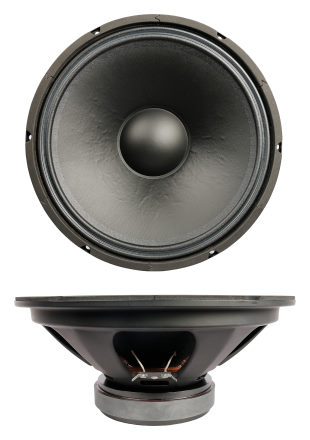open a bigger photo

recommended cabinet 1:
closed cabinet with 71 L volume
from 63/40 Hz (-3dB/-8dB)

recommended cabinet 2:
288 L volume bass reflex cabinet
with 2x HP100 reflex tube, 4 cm long.
from 29/25 Hz (-3dB/-8dB).

## SB Audience BIANCO-12OB150

order no. sba-b-12OB150

EUR 90,10excl. VAT: € 75.71 / \$ 81.01

30 cm bass driver with specially treated paper cone. The basket is made of die-cast.

• power handling (continuous/programme) = 150/300 W
• frequency range = 43-5000 Hz
• resonance frequency fs = 43 Hz
• impedance R = 8 Ohm
• sound pressure level SPL = 97 dB (1W; 1m)
• DC resistance Re = 7,2 Ohm
• force factor BL = 12,9 N/A
• voice coil inductance L = 1,14 mH
• effective piston radiating area Sd = 539,1 cm2
• effective mechanical mass incl. air load mms = 55,7 g
• equivalent volume of compliance Vas = 99,2 l
• total Q factor Qts = 0,54 (Qms=3,06, Qes=0,66)
• voice coil diameter = 60,5 mm
• maximum peak linear excursion vibration xlin = +/- 4,4 mm
• mounting diameter d = 276 mm
• overall diameter d = 305 mm
• mounting depth (not countersunk) t = 123 mm
• mass m = 3,6 kg
PDF data sheet

## 15 inch woofer## SB Audience BIANCO-15MW200

order no. sba-b-15MW200

EUR 98,40excl. VAT: € 82.69 / \$ 88.48

38 cm bass driver with specially treated paper cone.

• power handling (continuous/programme) = 200/400 W
• frequency range = 44-4250 Hz
• resonance frequency fs = 44 Hz
• impedance R = 8 Ohm
• sound pressure level SPL = 99 dB (1W; 1m)
• DC resistance Re = 6,8 Ohm
• force factor BL = 15,9 N/A
• voice coil inductance L = 1,02 mH
• effective piston radiating area Sd = 824,5 cm2
• effective mechanical mass incl. air load mms = 80,9 g
• equivalent volume of compliance Vas = 153,3 l
• total Q factor Qts = 0,59 (Qms=15,19, Qes=0,61)
• voice coil diameter = 60,5 mm
• maximum peak linear excursion vibration xlin = +/- 3,5 mm
• mounting diameter d = 350 mm
• overall diameter d = 384 mm
• mounting depth (not countersunk) t = 146,5 mm
• mass m = 5,12 kg
PDF data sheet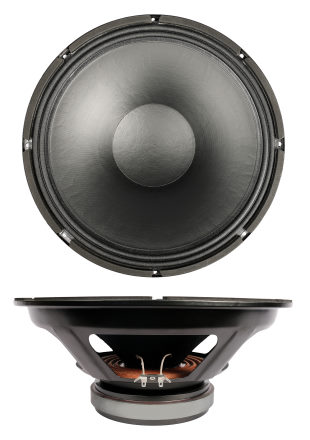open a bigger photo

recommended cabinet 1:
closed cabinet with 88 L volume
from 69/44 Hz (-3dB/-8dB)

recommended cabinet 2:
358 L volume bass reflex cabinet
with 4x HP100 reflex tube, 6 cm long.
from 31/27 Hz (-3dB/-8dB).

## SB Audience BIANCO-15W200

order no. sba-b-15W200

EUR 150,00excl. VAT: € 126.05 / \$ 134.87

38 cm bass driver with specially treated paper cone.

• power handling (continuous/programme) = 300/600 W
• frequency range = 47-4700 Hz
• resonance frequency fs = 47 Hz
• impedance R = 8 Ohm
• sound pressure level SPL = 99 dB (1W; 1m)
• DC resistance Re = 5,2 Ohm
• force factor BL = 17 N/A
• voice coil inductance L = 0,85 mH
• effective piston radiating area Sd = 907,9 cm2
• effective mechanical mass incl. air load mms = 107,1 g
• equivalent volume of compliance Vas = 123,5 l
• total Q factor Qts = 0,54 (Qms=10,62, Qes=0,57)
• voice coil diameter = 75,5 mm
• maximum peak linear excursion vibration xlin = +/- 5,5 mm
• mounting diameter d = 354 mm
• overall diameter d = 385,5 mm
• mounting depth (not countersunk) t = 153,5 mm
• mass m = 7,24 kg
PDF data sheet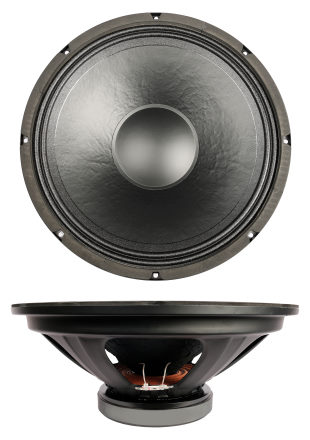open a bigger photo

recommended cabinet 1:
closed cabinet with 72 L volume
from 66/41 Hz (-3dB/-8dB)

recommended cabinet 2:
293 L volume bass reflex cabinet
with 2x HP100 reflex tube, 3 cm long.
from 31/26 Hz (-3dB/-8dB).

## SB Audience BIANCO-15MW350

order no. sba-b-15MW350

EUR 152,00excl. VAT: € 127.73 / \$ 136.67

38 cm bass driver with specially treated paper cone.

• power handling (continuous/programme) = 350/700 W
• frequency range = 39-3800 Hz
• resonance frequency fs = 39 Hz
• impedance R = 8 Ohm
• sound pressure level SPL = 101 dB (1W; 1m)
• DC resistance Re = 5,3 Ohm
• force factor BL = 16,9 N/A
• voice coil inductance L = 1,12 mH
• effective piston radiating area Sd = 855,3 cm2
• effective mechanical mass incl. air load mms = 108,7 g
• equivalent volume of compliance Vas = 157,5 l
• total Q factor Qts = 0,47 (Qms=13,09, Qes=0,49)
• voice coil diameter = 65,5 mm
• maximum peak linear excursion vibration xlin = +/- 5 mm
• mounting diameter d = 361 mm
• overall diameter d = 391 mm
• mounting depth (not countersunk) t = 156,6 mm
• mass m = 6,3 kg
PDF data sheet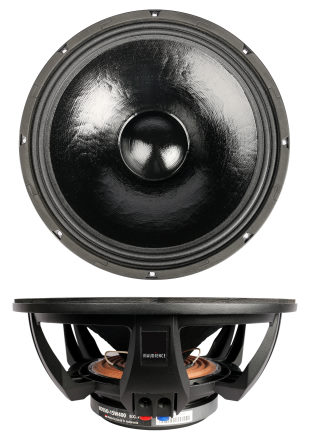open a bigger photo

recommended cabinet 1:
closed cabinet with 24 L volume
from 107/67 Hz (-3dB/-8dB)

recommended cabinet 2:
77 L volume bass reflex cabinet
with 2x HP100 reflex tube, 10 cm long.
from 57/44 Hz (-3dB/-8dB).

## SB Audience ROSSO-15W400

order no. sba-r-15W400

EUR 217,00excl. VAT: € 182.35 / \$ 195.12

39 cm bass driver with specially treated paper cone. The basket is made of die-cast.

• power handling (continuous/programme) = 400/800 W
• frequency range = 44-5200 Hz
• resonance frequency fs = 44 Hz
• impedance R = 8 Ohm
• sound pressure level SPL = 102 dB (1W; 1m)
• DC resistance Re = 5,2 Ohm
• force factor BL = 21,5 N/A
• voice coil inductance L = 1 mH
• effective piston radiating area Sd = 907,9 cm2
• effective mechanical mass incl. air load mms = 107,6 g
• equivalent volume of compliance Vas = 140,1 l
• total Q factor Qts = 0,33 (Qms=11,15, Qes=0,34)
• voice coil diameter = 75,5 mm
• maximum peak linear excursion vibration xlin = +/- 5 mm
• mounting diameter d = 361,4 mm
• overall diameter d = 394 mm
• mounting depth (not countersunk) t = 155,5 mm
• mass m = 8,7 kg
PDF data sheetopen a bigger photo

recommended cabinet 1:
closed cabinet with 22 L volume
from 99/62 Hz (-3dB/-8dB)

recommended cabinet 2:
74 L volume bass reflex cabinet
with 2x HP100 reflex tube, 14 cm long.
from 52/41 Hz (-3dB/-8dB).

## SB Audience ROSSO-15MW500

order no. sba-r-15MW500

EUR 235,00excl. VAT: € 197.48 / \$ 211.30

39 cm bass driver with specially treated paper cone. The basket is made of die-cast.

• power handling (continuous/programme) = 500/1000 W
• frequency range = 42-3700 Hz
• resonance frequency fs = 42 Hz
• impedance R = 8 Ohm
• sound pressure level SPL = 99 dB (1W; 1m)
• DC resistance Re = 5,6 Ohm
• force factor BL = 23,2 N/A
• voice coil inductance L = 1,37 mH
• effective piston radiating area Sd = 865,7 cm2
• effective mechanical mass incl. air load mms = 127,1 g
• equivalent volume of compliance Vas = 121,3 l
• total Q factor Qts = 0,34 (Qms=9,39, Qes=0,35)
• voice coil diameter = 99,2 mm
• maximum peak linear excursion vibration xlin = +/- 5,5 mm
• mounting diameter d = 361,5 mm
• overall diameter d = 394 mm
• mounting depth (not countersunk) t = 153,5 mm
• mass m = 11,2 kg
PDF data sheet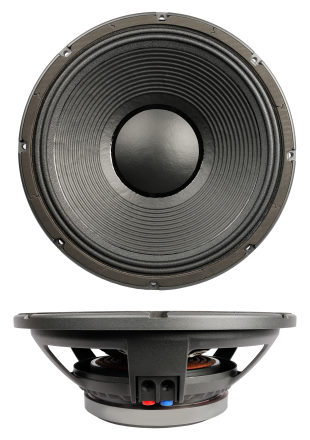open a bigger photo

recommended cabinet 1:
closed cabinet with 54 L volume
from 68/43 Hz (-3dB/-8dB)

recommended cabinet 2:
222 L volume bass reflex cabinet
with 2x HP100 reflex tube, 6 cm long.
from 31/26 Hz (-3dB/-8dB).

## SB Audience BIANCO-15SW400

order no. sba-b-15SW400

EUR 204,00excl. VAT: € 171.43 / \$ 183.43

39 cm bass driver with specially treated paper cone.

• power handling (continuous/programme) = 400/800 W
• frequency range = 41-2500 Hz
• resonance frequency fs = 41 Hz
• impedance R = 8 Ohm
• sound pressure level SPL = 98 dB (1W; 1m)
• DC resistance Re = 5,5 Ohm
• force factor BL = 19,8 N/A
• voice coil inductance L = 1,29 mH
• effective piston radiating area Sd = 845 cm2
• effective mechanical mass incl. air load mms = 134,1 g
• equivalent volume of compliance Vas = 111,5 l
• total Q factor Qts = 0,48 (Qms=22,45, Qes=0,49)
• voice coil diameter = 99,2 mm
• maximum peak linear excursion vibration xlin = +/- 6 mm
• mounting diameter d = 356 mm
• overall diameter d = 394 mm
• mounting depth (not countersunk) t = 133,5 mm
• mass m = 9,8 kg
PDF data sheetopen a bigger photo

recommended cabinet 1:
closed cabinet with 35 L volume
from 79/50 Hz (-3dB/-8dB)

recommended cabinet 2:
142 L volume bass reflex cabinet
with 2x HP100 reflex tube, 9 cm long.
from 37/31 Hz (-3dB/-8dB).

## SB Audience ROSSO-15SW800

order no. sba-r-15SW800

EUR 285,00excl. VAT: € 239.50 / \$ 256.26

39 cm bass driver with specially treated paper cone. The basket is made of die-cast.

• power handling (continuous/programme) = 800/1600 W
• frequency range = 45-4600 Hz
• resonance frequency fs = 45 Hz
• impedance R = 8 Ohm
• sound pressure level SPL = 99 dB (1W; 1m)
• DC resistance Re = 5,2 Ohm
• force factor BL = 21,1 N/A
• voice coil inductance L = 1,18 mH
• effective piston radiating area Sd = 850,1 cm2
• effective mechanical mass incl. air load mms = 143,6 g
• equivalent volume of compliance Vas = 87,6 l
• total Q factor Qts = 0,46 (Qms=9,99, Qes=0,48)
• voice coil diameter = 99,2 mm
• maximum peak linear excursion vibration xlin = +/- 8 mm
• mounting diameter d = 361,4 mm
• overall diameter d = 394 mm
• mounting depth (not countersunk) t = 168,5 mm
• mass m = 11,63 kg
PDF data sheet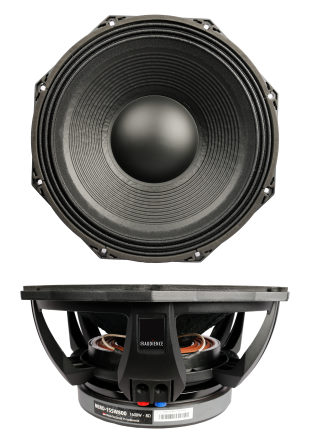open a bigger photo

recommended cabinet 1:
closed cabinet with 27 L volume
from 71/45 Hz (-3dB/-8dB)

recommended cabinet 2:
91 L volume bass reflex cabinet
with 2x HP100 reflex tube, 27 cm long.
from 37/29 Hz (-3dB/-8dB).

## SB Audience NERO-15SW800

order no. sba-n-15SW800

EUR 371,00excl. VAT: € 311.76 / \$ 333.59

39 cm bass driver with specially treated paper cone. The basket is made of die-cast.

• power handling (continuous/programme) = 800/1600 W
• frequency range = 31-2500 Hz
• resonance frequency fs = 31 Hz
• impedance R = 8 Ohm
• sound pressure level SPL = 97 dB (1W; 1m)
• DC resistance Re = 5,3 Ohm
• force factor BL = 24,4 N/A
• voice coil inductance L = 2,08 mH
• effective piston radiating area Sd = 860,5 cm2
• effective mechanical mass incl. air load mms = 207,2 g
• equivalent volume of compliance Vas = 137 l
• total Q factor Qts = 0,34 (Qms=7,59, Qes=0,36)
• voice coil diameter = 113 mm
• maximum peak linear excursion vibration xlin = +/- 10 mm
• mounting diameter d = 361,4 mm
• overall diameter d = 404 mm
• mounting depth (not countersunk) t = 177,7 mm
• mass m = 16,34 kg
PDF data sheet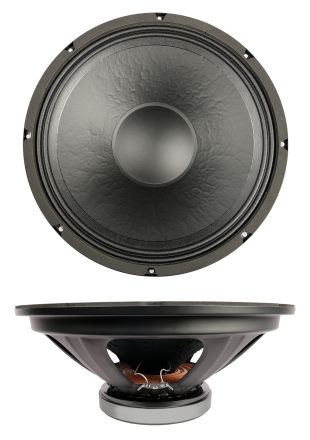open a bigger photo

recommended cabinet 1:
closed cabinet with 187 L volume
from 47/30 Hz (-3dB/-8dB)

recommended cabinet 2:
734 L volume bass reflex cabinet
with 4x HP100 reflex tube, 7 cm long.
from 21/19 Hz (-3dB/-8dB).

## SB Audience BIANCO-15OB350

order no. sba-b-15OB350

EUR 146,00excl. VAT: € 122.69 / \$ 131.28

38 cm bass driver with specially treated paper cone.

• power handling (continuous/programme) = 350/700 W
• frequency range = 34-3700 Hz
• resonance frequency fs = 34 Hz
• impedance R = 8 Ohm
• sound pressure level SPL = 98 dB (1W; 1m)
• DC resistance Re = 7,2 Ohm
• force factor BL = 16,48 N/A
• voice coil inductance L = 1,72 mH
• effective piston radiating area Sd = 855,3 cm2
• effective mechanical mass incl. air load mms = 106,48 g
• equivalent volume of compliance Vas = 212,73 l
• total Q factor Qts = 0,57 (Qms=12,17, Qes=0,6)
• voice coil diameter = 65,5 mm
• maximum peak linear excursion vibration xlin = +/- 8,5 mm
• mounting diameter d = 361 mm
• overall diameter d = 391 mm
• mounting depth (not countersunk) t = 168,5 mm
• mass m = 6 kg
PDF data sheet

## 18 inch woofer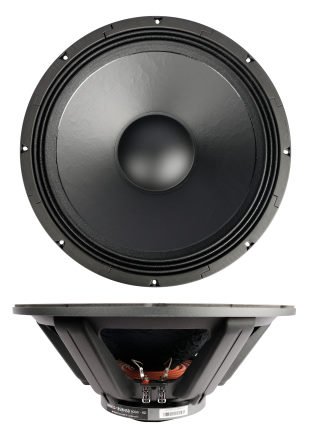open a bigger photo

recommended cabinet 1:
closed cabinet with 140 L volume
from 50/32 Hz (-3dB/-8dB)

recommended cabinet 2:
558 L volume bass reflex cabinet
with 4x HP100 reflex tube, 13 cm long.
from 24/20 Hz (-3dB/-8dB).

## SB Audience BIANCO-18SW450

order no. sba-b-18SW450

EUR 219,00excl. VAT: € 184.03 / \$ 196.92

46 cm bass driver with specially treated paper cone.

• power handling (continuous/programme) = 450/900 W
• frequency range = 28-3000 Hz
• resonance frequency fs = 28 Hz
• impedance R = 8 Ohm
• sound pressure level SPL = 102 dB (1W; 1m)
• DC resistance Re = 5 Ohm
• force factor BL = 18,8 N/A
• voice coil inductance L = 1,37 mH
• effective piston radiating area Sd = 1244,1 cm2
• effective mechanical mass incl. air load mms = 190,3 g
• equivalent volume of compliance Vas = 370,4 l
• total Q factor Qts = 0,45 (Qms=8,39, Qes=0,47)
• voice coil diameter = 75,5 mm
• maximum peak linear excursion vibration xlin = +/- 8 mm
• mounting diameter d = 426 mm
• overall diameter d = 470 mm
• mounting depth (not countersunk) t = 189,5 mm
• mass m = 9,05 kg
PDF data sheet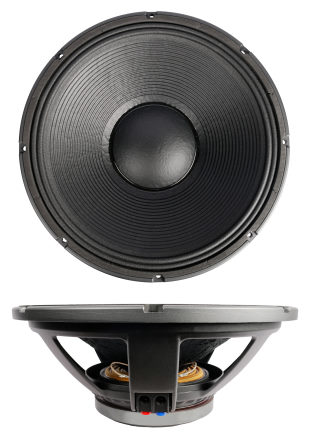open a bigger photo

recommended cabinet 1:
closed cabinet with 197 L volume
from 47/29 Hz (-3dB/-8dB)

recommended cabinet 2:
808 L volume bass reflex cabinet
with 4x HP100 reflex tube, 7 cm long.
from 21/18 Hz (-3dB/-8dB).

## SB Audience BIANCO-18SW500

order no. sba-b-18SW500

EUR 245,00excl. VAT: € 205.88 / \$ 220.29

46 cm bass driver with specially treated paper cone.

• power handling (continuous/programme) = 500/1000 W
• frequency range = 30-3100 Hz
• resonance frequency fs = 30 Hz
• impedance R = 8 Ohm
• sound pressure level SPL = 101 dB (1W; 1m)
• DC resistance Re = 5,7 Ohm
• force factor BL = 19,8 N/A
• voice coil inductance L = 1,64 mH
• effective piston radiating area Sd = 1269,2 cm2
• effective mechanical mass incl. air load mms = 195,2 g
• equivalent volume of compliance Vas = 334,8 l
• total Q factor Qts = 0,52 (Qms=19,04, Qes=0,53)
• voice coil diameter = 99,2 mm
• maximum peak linear excursion vibration xlin = +/- 6 mm
• mounting diameter d = 422 mm
• overall diameter d = 465 mm
• mounting depth (not countersunk) t = 174 mm
• mass m = 10,28 kg
PDF data sheet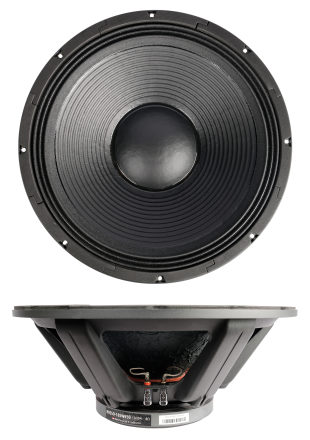open a bigger photo

recommended cabinet 1:
closed cabinet with 95 L volume
from 59/37 Hz (-3dB/-8dB)

recommended cabinet 2:
366 L volume bass reflex cabinet
with 4x HP100 reflex tube, 15 cm long.
from 29/24 Hz (-3dB/-8dB).

## SB Audience ROSSO-18SW650

order no. sba-r-18SW650

EUR 297,00excl. VAT: € 249.58 / \$ 267.05

46 cm bass driver with specially treated paper cone. The basket is made of die-cast.

• power handling (continuous/programme) = 650/1300 W
• frequency range = 31-3200 Hz
• resonance frequency fs = 31 Hz
• impedance R = 8 Ohm
• sound pressure level SPL = 101 dB (1W; 1m)
• DC resistance Re = 5 Ohm
• force factor BL = 20,6 N/A
• voice coil inductance L = 1,4 mH
• effective piston radiating area Sd = 1244,1 cm2
• effective mechanical mass incl. air load mms = 189,7 g
• equivalent volume of compliance Vas = 304,3 l
• total Q factor Qts = 0,42 (Qms=8,32, Qes=0,44)
• voice coil diameter = 99,2 mm
• maximum peak linear excursion vibration xlin = +/- 8,5 mm
• mounting diameter d = 426 mm
• overall diameter d = 470 mm
• mounting depth (not countersunk) t = 195,5 mm
• mass m = 11,65 kg
PDF data sheet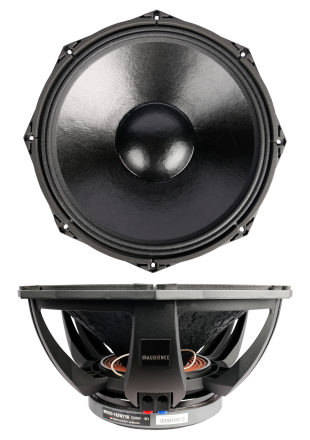open a bigger photo

recommended cabinet 1:
closed cabinet with 73 L volume
from 66/42 Hz (-3dB/-8dB)

recommended cabinet 2:
290 L volume bass reflex cabinet
with 4x HP100 reflex tube, 16 cm long.
from 32/26 Hz (-3dB/-8dB).

## SB Audience ROSSO-18SW750

order no. sba-r-18SW750

EUR 324,00excl. VAT: € 272.27 / \$ 291.33

47 cm bass driver with specially treated paper cone. The basket is made of die-cast.

• power handling (continuous/programme) = 750/1500 W
• frequency range = 36-4100 Hz
• resonance frequency fs = 36 Hz
• impedance R = 8 Ohm
• sound pressure level SPL = 101 dB (1W; 1m)
• DC resistance Re = 5,1 Ohm
• force factor BL = 23,3 N/A
• voice coil inductance L = 1,59 mH
• effective piston radiating area Sd = 1256,6 cm2
• effective mechanical mass incl. air load mms = 212,4 g
• equivalent volume of compliance Vas = 206,9 l
• total Q factor Qts = 0,43 (Qms=10,5, Qes=0,45)
• voice coil diameter = 113 mm
• maximum peak linear excursion vibration xlin = +/- 5 mm
• mounting diameter d = 428 mm
• overall diameter d = 483 mm
• mounting depth (not countersunk) t = 213,5 mm
• mass m = 16,34 kg
PDF data sheetopen a bigger photo

recommended cabinet 1:
closed cabinet with 104 L volume
from 58/37 Hz (-3dB/-8dB)

recommended cabinet 2:
418 L volume bass reflex cabinet
with 4x HP100 reflex tube, 13 cm long.
from 27/23 Hz (-3dB/-8dB).

## SB Audience ROSSO-18SW800

order no. sba-r-18SW800

EUR 303,00excl. VAT: € 254.62 / \$ 272.45

46 cm bass driver with specially treated paper cone. The basket is made of die-cast.

• power handling (continuous/programme) = 800/1600 W
• frequency range = 33-3200 Hz
• resonance frequency fs = 33 Hz
• impedance R = 8 Ohm
• sound pressure level SPL = 100 dB (1W; 1m)
• DC resistance Re = 5,1 Ohm
• force factor BL = 21 N/A
• voice coil inductance L = 1,25 mH
• effective piston radiating area Sd = 1256,6 cm2
• effective mechanical mass incl. air load mms = 200,6 g
• equivalent volume of compliance Vas = 258,1 l
• total Q factor Qts = 0,46 (Qms=9,09, Qes=0,48)
• voice coil diameter = 99,2 mm
• maximum peak linear excursion vibration xlin = +/- 7,5 mm
• mounting diameter d = 425 mm
• overall diameter d = 470 mm
• mounting depth (not countersunk) t = 205,5 mm
• mass m = 12,7 kg
PDF data sheetopen a bigger photo

recommended cabinet 1:
closed cabinet with 70 L volume
from 60/38 Hz (-3dB/-8dB)

recommended cabinet 2:
285 L volume bass reflex cabinet
with 4x HP100 reflex tube, 23 cm long.
from 28/24 Hz (-3dB/-8dB).

## SB Audience ROSSO-18SW1000D

order no. sba-r-18SW1000D

EUR 383,00excl. VAT: € 321.85 / \$ 344.38

47 cm bass driver with specially treated paper cone. The basket is made of die-cast.

• power handling (continuous/programme) = 1000/2000 W
• frequency range = 35-2900 Hz
• resonance frequency fs = 35 Hz
• impedance R = 8 Ohm
• sound pressure level SPL = 99 dB (1W; 1m)
• DC resistance Re = 5,3 Ohm
• force factor BL = 25,8 N/A
• voice coil inductance L = 1,64 mH
• effective piston radiating area Sd = 1256,6 cm2
• effective mechanical mass incl. air load mms = 276 g
• equivalent volume of compliance Vas = 164,3 l
• total Q factor Qts = 0,47 (Qms=10,13, Qes=0,49)
• voice coil diameter = 113 mm
• maximum peak linear excursion vibration xlin = +/- 10 mm
• mounting diameter d = 428 mm
• overall diameter d = 483 mm
• mounting depth (not countersunk) t = 218,7 mm
• mass m = 17 kg
PDF data sheetopen a bigger photo

recommended cabinet 1:
closed cabinet with 26 L volume
from 87/55 Hz (-3dB/-8dB)

recommended cabinet 2:
84 L volume bass reflex cabinet
with 2x HP100 reflex tube, 17 cm long.
from 47/36 Hz (-3dB/-8dB).

## SB Audience NERO-18SW1100D

order no. sba-n-18SW1100D

EUR 409,00excl. VAT: € 343.70 / \$ 367.76

47 cm bass driver with specially treated paper cone. The basket is made of die-cast.

• power handling (continuous/programme) = 1100/2200 W
• frequency range = 36-3000 Hz
• resonance frequency fs = 36 Hz
• impedance R = 8 Ohm
• sound pressure level SPL = 101 dB (1W; 1m)
• DC resistance Re = 5 Ohm
• force factor BL = 30,9 N/A
• voice coil inductance L = 1,36 mH
• effective piston radiating area Sd = 1256,6 cm2
• effective mechanical mass incl. air load mms = 294 g
• equivalent volume of compliance Vas = 153,1 l
• total Q factor Qts = 0,33 (Qms=11,83, Qes=0,34)
• voice coil diameter = 127 mm
• maximum peak linear excursion vibration xlin = +/- 9 mm
• mounting diameter d = 426 mm
• overall diameter d = 483 mm
• mounting depth (not countersunk) t = 212,7 mm
• mass m = 20,7 kg
PDF data sheet

## 21 inch woofer## SB Audience ROSSO-21SW800

order no. sba-r-21SW800

EUR 516,00excl. VAT: € 433.61 / \$ 463.97

53 cm bass driver with specially treated paper cone. The basket is made of die-cast.

• power handling (continuous/programme) = 800/1600 W
• frequency range = 38-3200 Hz
• resonance frequency fs = 38 Hz
• impedance R = 8 Ohm
• sound pressure level SPL = 100 dB (1W; 1m)
• DC resistance Re = 6,4 Ohm
• force factor BL = 24,1 N/A
• voice coil inductance L = 1,82 mH
• effective piston radiating area Sd = 1661,9 cm2
• effective mechanical mass incl. air load mms = 301,4 g
• equivalent volume of compliance Vas = 224,4 l
• total Q factor Qts = 0,76 (Qms=14,41, Qes=0,8)
• voice coil diameter = 113 mm
• maximum peak linear excursion vibration xlin = +/- 7,5 mm
• mounting diameter d = 490 mm
• overall diameter d = 540 mm
• mounting depth (not countersunk) t = 230 mm
• mass m = 16,7 kg
PDF data sheet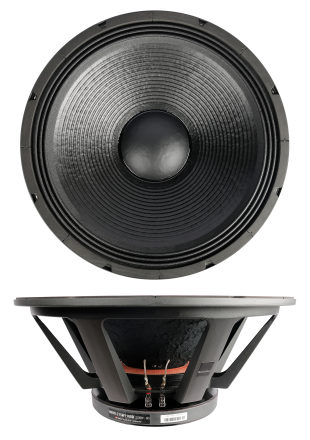open a bigger photo

recommended cabinet 1:
closed cabinet with 57 L volume
from 75/48 Hz (-3dB/-8dB)

recommended cabinet 2:
190 L volume bass reflex cabinet
with 4x HP100 reflex tube, 22 cm long.
from 40/31 Hz (-3dB/-8dB).

## SB Audience NERO-21SW1100D

order no. sba-n-21SW1100D

EUR 521,00excl. VAT: € 437.82 / \$ 468.46

53 cm bass driver with specially treated paper cone. The basket is made of die-cast.

• power handling (continuous/programme) = 1100/2200 W
• frequency range = 32-2600 Hz
• resonance frequency fs = 32 Hz
• impedance R = 8 Ohm
• sound pressure level SPL = 104 dB (1W; 1m)
• DC resistance Re = 5,1 Ohm
• force factor BL = 30 N/A
• voice coil inductance L = 1,29 mH
• effective piston radiating area Sd = 1654,7 cm2
• effective mechanical mass incl. air load mms = 309,3 g
• equivalent volume of compliance Vas = 312,6 l
• total Q factor Qts = 0,34 (Qms=10,92, Qes=0,35)
• voice coil diameter = 127 mm
• maximum peak linear excursion vibration xlin = +/- 9 mm
• mounting diameter d = 490 mm
• overall diameter d = 540 mm
• mounting depth (not countersunk) t = 233,2 mm
• mass m = 21 kg
PDF data sheet

## Coaxial speaker## SB Audience BIANCO-8CX100

order no. sba-b-8CX100

20 cm coax driver with specially treated paper cone.

• power handling (continuous/programme) = 100/200 W
• frequency range = 87-20000 Hz
• resonance frequency fs = 87 Hz
• impedance R = 8 Ohm
• sound pressure level SPL = 92 dB (1W; 1m)
• DC resistance Re = 5 Ohm
• force factor BL = 7,9 N/A
• voice coil inductance L = 0,64 mH
• effective piston radiating area Sd = 216 cm2
• effective mechanical mass incl. air load mms = 22,1 g
• equivalent volume of compliance Vas = 10 l
• total Q factor Qts = 0,8 (Qms=4,54, Qes=0,97)
• voice coil diameter = 49,5 mm
• maximum peak linear excursion vibration xlin = +/- 7 mm
• mounting diameter d = 179,6 mm
• overall diameter d = 205,6 mm
• mounting depth (not countersunk) t = 117 mm
• mass m = 3,3 kg
PDF data sheet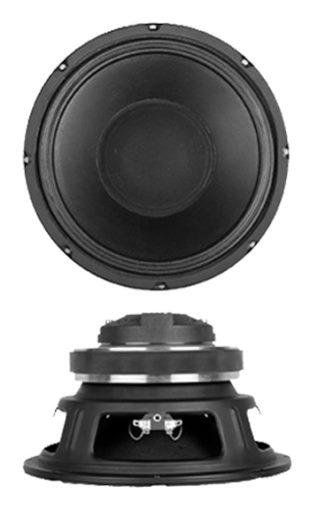open a bigger photo

recommended cabinet 1:
closed cabinet with 18 L volume
from 92/58 Hz (-3dB/-8dB)

recommended cabinet 2:
76 L volume bass reflex cabinet
with HP100 reflex tube, 7 cm long.
from 42/36 Hz (-3dB/-8dB).

## SB Audience BIANCO-10CX150

order no. sba-b-10CX150

25 cm coax driver with specially treated paper cone.

• power handling (continuous/programme) = 150/300 W
• frequency range = 58-20000 Hz
• resonance frequency fs = 58 Hz
• impedance R = 8 Ohm
• sound pressure level SPL = 95 dB (1W; 1m)
• DC resistance Re = 5,2 Ohm
• force factor BL = 11,8 N/A
• voice coil inductance L = 0,6 mH
• effective piston radiating area Sd = 353 cm2
• effective mechanical mass incl. air load mms = 38 g
• equivalent volume of compliance Vas = 33,4 l
• total Q factor Qts = 0,5 (Qms=9,24, Qes=0,53)
• voice coil diameter = 60,5 mm
• maximum peak linear excursion vibration xlin = +/- 5 mm
• mounting diameter d = 229 mm
• overall diameter d = 258 mm
• mounting depth (not countersunk) t = 137,2 mm
• mass m = 4,96 kg
PDF data sheet

## SB Audience BIANCO-12CX200

order no. sba-b-12CX200

30 cm coax driver with specially treated paper cone.

• power handling (continuous/programme) = 200/400 W
• frequency range = 60-20000 Hz
• resonance frequency fs = 50 Hz
• impedance R = 8 Ohm
• sound pressure level SPL = 96 dB (1W; 1m)
• DC resistance Re = 5,2 Ohm
• force factor BL = 11,2 N/A
• voice coil inductance L = 0,7 mH
• effective piston radiating area Sd = 540 cm2
• effective mechanical mass incl. air load mms = 46,5 g
• equivalent volume of compliance Vas = 99 l
• total Q factor Qts = 0,59 (Qms=9,27, Qes=0,63)
• voice coil diameter = 60,5 mm
• maximum peak linear excursion vibration xlin = +/- 5 mm
• mounting diameter d = 278 mm
• overall diameter d = 306 mm
• mounting depth (not countersunk) t = 149 mm
• mass m = 5,26 kg
PDF data sheet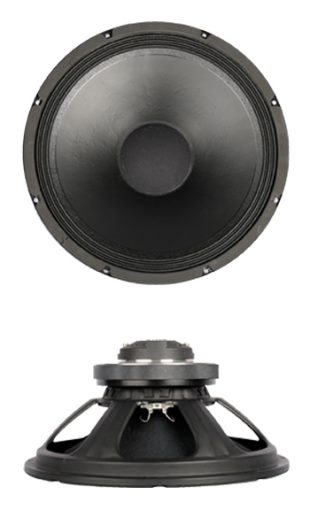open a bigger photo

recommended cabinet 1:
closed cabinet with 114 L volume
from 61/38 Hz (-3dB/-8dB)

recommended cabinet 2:
467 L volume bass reflex cabinet
with 4x HP100 reflex tube, 6 cm long.
from 28/24 Hz (-3dB/-8dB).

## SB Audience BIANCO-15CX250

order no. sba-b-15CX250

38 cm coax driver with specially treated paper cone.

• power handling (continuous/programme) = 250/500 W
• frequency range = 38-19500 Hz
• resonance frequency fs = 37,5 Hz
• impedance R = 4 Ohm
• sound pressure level SPL = 103 dB (1W; 1m)
• DC resistance Re = 2,8 Ohm
• force factor BL = 10,8 N/A
• voice coil inductance L = 0,58 mH
• effective piston radiating area Sd = 881,4 cm2
• effective mechanical mass incl. air load mms = 89 g
• equivalent volume of compliance Vas = 219,7 l
• total Q factor Qts = 0,48 (Qms=9,57, Qes=0,5)
• voice coil diameter = 75,5 mm
• maximum peak linear excursion vibration xlin = +/- 7 mm
• mounting diameter d = 361 mm
• overall diameter d = 391 mm
• mounting depth (not countersunk) t = 176 mm
• mass m = 6,75 kg
PDF data sheet

## no longer sold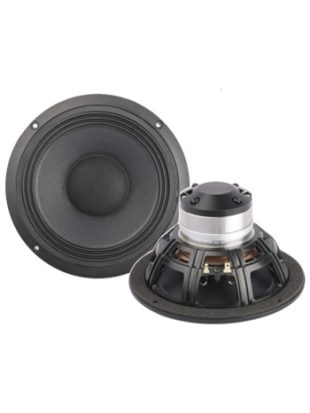open a bigger photo

recommended cabinet 1:
closed cabinet with 2,5 L volume
from 147/93 Hz (-3dB/-8dB)

recommended cabinet 2:
10,2 L volume bass reflex cabinet
with HP70 reflex tube, 13 cm long.
from 70/58 Hz (-3dB/-8dB).

## SB Audience BIANCO-6CXN80

order no. not-available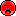- not available

17 cm coax driver with specially treated paper cone.

• power handling (continuous/programme) = 80/160 W
• frequency range = 84-20000 Hz
• resonance frequency fs = 84 Hz
• impedance R = 8 Ohm
• sound pressure level SPL = 92 dB (1W; 1m)
• DC resistance Re = 5,2 Ohm
• force factor BL = 8,8 N/A
• voice coil inductance L = 0,45 mH
• effective piston radiating area Sd = 134,8 cm2
• effective mechanical mass incl. air load mms = 14,4 g
• equivalent volume of compliance Vas = 6,3 l
• total Q factor Qts = 0,46 (Qms=4,22, Qes=0,51)
• voice coil diameter = 38,5 mm
• maximum peak linear excursion vibration xlin = +/- 3,75 mm
• mounting diameter d = 146,5 mm
• overall diameter d = 176 mm
• mounting depth (not countersunk) t = 93,9 mm
• mass m = 0,98 kg
PDF data sheet

The cabinet volume calculations are executed considering an external resistance of 0.3 Ohm. We are using, if no other data is known or available, the information supplied by the manufacturer.

Please note: the frequency response doesn't say much about the cut-off frequency inside the cabinet! Therefore, we mostly give you 2 examples with dimensioning considerations and an indication, how low the loudspeaker will reproduce.

Home